# NCERT Solutions For Class 10 Maths Chapter 12 Exercise 12.2

Go back to  'Areas Related to Circles'

## Chapter 12 Ex.12.2 Question 1

Find the area of a sector of a circle with radius $$6\,\rm{cm}$$ if angle of the sector is $${60^\circ}$$.

### Solution

What is known?

A circle with radius $$= 6 \,\rm{cm,}$$ angle of the sector $$= {60^\circ}$$

What is unknown?

Area of sector of a circle.

Reasoning:

The formula for Area of the sector of angle $$\rm{\theta}$$

\begin{align}= \frac{\theta }{{360}} \times \pi {r^2}\end{align}

Steps:

$$\rm{\theta} = {60^\circ }$$ Radius $$= 6\rm{}\,cm$$

Area of the sector

\begin{align}&= \frac{\theta }{{360}} \times \pi {r^2}\\ &= \frac{{{{60}^o}}}{{{{360}^o}}} \times \frac{{22}}{7} \times 6 \times 6\\ &= \frac{{132}}{7}{\text{c}}{{\text{m}}^2}\\ &= 18\frac{6}{7}{\text{ c}}{{\text{m}}^2}\end{align}

## Chapter 12 Ex.12.2 Question 2

Find the area of a quadrant of a circle whose circumference is $$22\, \rm{cm.}$$

### Solution

What is known?

A circle whose circumference is $$22\, \rm{cm.}$$

What is unknown?

Area of quadrant of a circle.

Reasoning:

Find the radius of the circle $$(r)$$ from the circumference $$(c),$$ $${\bf{C}} = 2\pi r$$

Therefore, \begin{align} r = \frac{c}{{2\pi }}\end{align}

Since quadrant means one of the four equal parts. Using unitary method, since four quadrants corresponds to Area of a circle

\begin{align}&= \frac{1}{4} \times {\text{Area of a circle}}\\&= \frac{1}{4} \times \pi {r^2}\end{align}

Steps:

Circumference \begin{align}\left( c \right) = 22{\text{ cm}}\end{align}

Therefore,radius \begin{align}\rm{r} = \frac{C}{{2\pi }}\end{align}

\begin{align}&= \frac{{22}}{{2 \times \frac{{22}}{7}}}\\&= \frac{{22 \times 7}}{{2 \times 22}}\\
&= \frac{7}{2}\,\,\rm cm\end{align}

Therefore,Area of a quadrant \begin{align}= \frac{1}{4} \times \pi {r^2}\end{align}

\begin{align}&= \frac{1}{4} \times \frac{{22}}{7} \times \frac{7}{2} \times \frac{7}{2}\rm c{m^2}\\ &= \frac{{77}}{8}\rm c{m^2}\end{align}

## Chapter 12 Ex.12.2 Question 3

The length of the minute hand of a clock is $$14\, \rm{cm.}$$ Find the area swept by the minute hand in $$5$$ minutes.

### Solution

What is known?

Length of the minute hand of the clock

What is unknown?

Area swept by the minute hand in $$5$$ minutes.

Reasoning:

Since the minute hand completes one rotation in $$1$$ hour or $$60$$ minutes

Therefore,Area swept by the minute hand in $$60$$ minutes $$=$$  Area of the Circle with radius equal to the length of the minute hand \begin{align}= \pi {{r^2}}.\end{align}

Using Unitary method

Area swept by minute hand in $$1$$ minute: \begin{align}\frac{{\pi {r^2}}}{{60}}\end{align}

Area swept by minute hand in $$5$$ minutes: \begin{align}\frac{{\pi {r^2}}}{{60}} \times 5 = \frac{{\pi {r^2}}}{{12}}\end{align}

Steps:

Length of the minute hand $$({r}) = 14\,\rm{cm}$$

We know that the minute hand completes one rotation in $$1$$hour or $$60$$ minutes

Therefore,Area swept by the minute hand in $$60$$ minutes $$=$$ Area of circlewith radius '$$r$$'

\begin{align} &= \pi {r^2}\\& = \frac{{22}}{7} \times 14 \times 14\\ &= 22 \times 28{\text{c}}{{\text{m}}^2}\\ &= 616\;{\text{cm}^2}\end{align}

Therefore,Area swept by the minute hand in $$5$$ minutes =\begin{align}\frac{5}{{60}}\pi {r^2} \Rightarrow \frac{1}{{12}}\pi {r^2}\end{align}

\begin{align}&= \frac{1}{{12}} \times \frac{{22}}{7} \times 14 \times 14\rm c{m^2}\\&= \frac{{154}}{3}\rm c{m^2} \end{align}

## Chapter 12 Ex.12.2 Question 4

A chord of a circle of radius $$10\, \rm{cm}$$ subtends a right angle at the centre. Find the area of the corresponding

(i) minor segment

(ii) major sector. (Use $$\pi = 3.14$$)

### Solution

What is known?

Radius of the circle and angle subtended by the chord at the center.

What is unknown?

(i) Area of minor segment

(ii) Area of major segment

Reasoning:

In a circle with radius r and angle at the centre with degree measure $$\theta$$;

(i) Area of the sector\begin{align}= \frac{{\rm{\theta }}}{{360}^{\rm{o}}} \times \pi {r^2}\end{align}

(ii) Area of the segment = Area of the sector- Area of the corresponding triangle.

Area of the triangle\begin{align}= \frac{1}{2} \times \rm base \times height\end{align}

Draw a figure to visualize the area to be found out.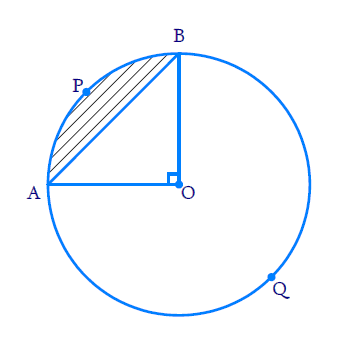Here,\begin{align}{\rm{Radius, }}r = 10\rm \,cm{\rm{\theta }} = {90^{\rm{o}}}\end{align}

Visually it's clear from the figure that;

Let $${AB}$$ is the chord subtends a right angle at the centre.

(i) Area of minor segment $${APB} =$$ $$\text{Area of sector}$$ $${OAPB}\, -\,$$$$\text{Area of right}$$$$\Delta {AOB}$$

(ii) Area of major segment \begin{align}AQB{\rm{ }} = \pi {r^2} - \text{Area of minor segment APB}\end{align}

Area of the right triangle

\begin{align}\Delta AOB = \frac{1}{2} \times OA \times OB\end{align}

\begin{align}&= {\frac{\theta }{{{{360}^\circ }}} \times \pi {r^2}}\\&= {\frac{{{{90}^\circ }}}{{{{360}^\circ }}} \times \pi {r^2}}\\&= {\frac{{\pi {r^2}}}{4}}\end{align}

\begin{align} &= \frac{1}{2} \times {\text{ base }} \times \,\text{height}\\ &= \frac{1}{2} \times {OA} \times {OB}\,\,\,\,\,\,\,\,\,\,\left( {\because {{OA}} \bot {{OB}}} \right)\\& = \frac{1}{2} \times {\text{radius}} \times \rm{radius}\end{align}

Steps:(i) Area of the minor segment $${APB}$$$$=$$Area of sector \begin{align}\rm{OAPB} - \text{ Area of right }{\Delta AOB}\end{align}

\begin{align} & =\left[ \begin{array} & \frac{{{90}^{{}^\circ }}}{{{360}^{{}^\circ }}}\times \pi {{r}^{2}}- \\ \frac{1}{2}\times OA\times OB \\ \end{array} \right]\ \\ & (\because OA\bot OB) \\ & =\frac{\pi {{\text{r}}^{\text{2}}}}{4}-\frac{1}{2}\times r\times r \\ & ={{\text{r}}^{\text{2}}}\left( \frac{\pi }{\text{4}}\text{-}\frac{\text{1}}{\text{2}} \right) \\ & ={{r}^{2}}\left( \frac{3.14-2}{4} \right) \\ & =\left( \frac{{{r}^{2}}\times 1.14}{4} \right) \\ & =\frac{10\times 10\times 1.14}{4}\text{ c}{{\text{m}}^{2}} \\ & =28.5\text{c}{{\text{m}}^{2}} \\ \end{align}

ii) Area of major segment $${AQB} =\pi \rm{r^2}-$$ Area of minor segment $${APB}$$

\begin{align} &= \pi \times 10 \times 10 - 28.5\\&= 3.14 \times 100 - 28.5\\&= 314 - 28.5\\&= 285.5{\text{ cm}^2}\end{align}

## Chapter 12 Ex.12.2 Question 5

In a circle of radius $$21\,\rm {cm,}$$ an arc subtends an angle of $$60^\circ$$ at the centre. Find:

(i) The length of the arc

(ii) Area of the sector formed by the arc

(iii) Area of the segment formed by the corresponding chord

### Solution

What is known?

Radius of the circle and angle subtended by the arc at the center.

What is unknown?

(i) Length of the arc

(ii) Area of the sector formed by the arc

(iii) Area of the segment formed by the corresponding chord

Reasoning:

In a circle with radius r and angle at the center with degree measure $$\theta$$;

(i) Length of the arc\begin{align}&= \frac{{\rm{\theta }}}{{360}^{\rm{o}}} \times 2\pi {r}\end{align}

(ii) Area of the sector\begin{align} = \frac{{\rm{\theta }}}{{360}^{\rm{o}}} \times \pi {r^2}\end{align}

(iii) Area of the segment = Area of the sector- Area of the corresponding triangle

Draw a figure to visualize the problem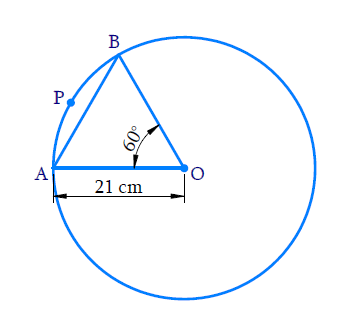Here, $$\rm{}r= 21\,cm$$$$\theta = {60^\circ}$$

\begin{align} &= {\frac{\theta }{{{{360}^\circ }}} \times 2\pi r}\\&{ = \frac{{2\pi r}}{6}}\end{align}

\begin{align}&{ = \frac{\theta }{{{{360}^\circ }}} \times \pi {r^2}}\\&{ = \frac{{\pi {r^2}}}{6}}\end{align}Visually it’s clear from the figure that;

Area of the segment $${APB} =$$Area of sector $${OAPB} -\,$$Area of $$\Delta {OAB}$$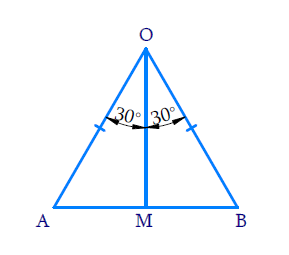Draw $${OM}\, \bot \,{AB}$$

Now consider$$\Delta {OAM}$$ and $$\Delta {OBM}$$

$$OA\,=\,OB$$(radii of a circle)

$${OM}\,= \,{OM}$$(Common)

\begin{align} &\angle {{OMA}}\!=\!\!\angle {{OMB}}\!=\!{90^ \circ }\left(\because\!{\,{{OM}} \bot {{AB}}} \!\right)\\ \end{align}

$$\therefore \,\,\Delta {{OAM}} \cong {{\Delta OBM}}$$(By RHS congruency)

\begin{align}\begin{bmatrix}{\cos {30}^\circ } = \frac{{OM}}{{OA}} \\ {\text{ or }} \\ \text{OM}= {\text{r}}\cos {{30}^\circ } = \frac{{r\sqrt 3 }}{2}\end{bmatrix}\end{align}

\begin{align}{AM} &= {r \times \frac{1}{2} = \frac{r}{2}}\end{align}

$$\text{Area of}\,\Delta OAB$$\begin{align}&= \frac{1}{2} \times AB \times OM\\&= \frac{{\sqrt 3 }}{4}{r^2}\end{align}

Steps:Radius $${(r)} = 21 \,{cm}$$ $$\theta = {60^o}$$

i) Length of the arc APB

\begin{align}&= \frac{\theta }{{{{360}^o}}} \times 2\pi r\\ &= \frac{{{{60}^\circ }}}{{{{360}^\circ }}} \times 2 \times \frac{{22}}{7} \times 21\rm{cm}\\&= 22\,{\text{cm}}\end{align}

ii) Area of the sector, AOBP

\begin{align}& = \frac{\theta }{{{{360}^o}}} \times \pi {r^2}\\ &= \frac{{{{60}^o}}}{{{{360}^o}}} \times \frac{{22}}{7} \times 21 \times 21\rm{cm^2}\\&= 231\,{\text{c}}{{\text{m}}^2}\end{align}

iii) Area of the segment = Area of the sector - area of the triangle AOB

To find area of the segment, we need to find thearea of $$\Delta {OAB}$$Draw $$OM \bot AB$$

Consider $$\Delta OAM{\text{ and }}\Delta OMB$$

$$\angle {OMA} = \angle {OMB} = {90^o}$$ (Since $${OM} \,\bot\, {AB}$$)

Therefore $$\Delta OMB \cong \Delta OMA$$(By RHS Congruency)

So, $${AM} = {MB}$$ (Corresponding parts of congruent triangles)

\begin{align}\angle OMB = \angle OMA = \frac{1}{2} \times {60^o} = {30^o}\end{align}

In $$\Delta {AOM}$$

\begin{align}{\cos {{30}^\circ } }&{= \frac{{OM}}{{OA}}{\text{ or OM}} = r\frac{{\sqrt 3 }}{2}}\\{\sin {{30}^\circ }}&\!\!=\! {\frac{{AM}}{{OA}}{\text{ or AM}}\!=\!\! \frac{1}{2}\!\times\! r\!=\!\!\frac{r}{2}}\\{ \Rightarrow \quad{\text{AB}}}&= {2{\text{AM}} = 2\frac{r}{2} = r}\end{align}

\begin{align}{\rm{AB}} &= 2{\rm{AM}}\\AB &= 2 \times \frac{1}{2}r\\AB &= r\end{align}

Therefore, Area of $$\Delta {AOB}$$

\begin{align}&= \frac{1}{2} \times AB \times OM\\& = {\frac{1}{2} \times {r} \times \frac{{r\sqrt 3 }}{2}}\\& = {\frac{{21 \times 21\sqrt 3 }}{4}}\\ &={ \frac{{441\sqrt 3 }}{4}{\text{c}}{{\text{m}}^2}}\end{align}

Area of the segment

\begin{align}= \left( {231 - \frac{{441\sqrt 3 }}{4}} \right)c{m^2}\end{align}

## Chapter 12 Ex.12.2 Question 6

A chord of a circle of radius $$15\, \rm{cm}$$ subtends an angle of $$60^\circ$$ at the Centre. Find the areas of the corresponding minor and major segments of the circle.

(Use $$\pi ={ }3.14$$ and  $$\sqrt{3}=1.73$$)

### Solution

What is known?

A chord of a circle with radius $${(r)} = 15\, \rm{cm}$$ subtends an angle \begin{align}(\theta)=60^{\circ}\end{align} at the centre.

What is unknown?

Area of minor and major segments of the circle.

Reasoning:

In a circle with radius r and angle at the centre with degree measure $$\rm{\theta }$$;

(i) Area of the sector \begin{align} = \frac{{\rm{\theta }}}{360}^{\rm{o}} \times \pi {r^2}\end{align}

(ii) Area of the segment = Area of the sector - Area of the corresponding triangle

Draw a figure to visualize the area to be found out.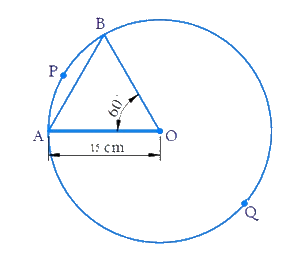Here, \begin{align}\rm{Radius, }r =15\,cm\,\,\,\,\,\,\,\,\,\,{\rm{ \theta }} = {60^{\rm{\circ}}}\end{align}

Visually it's clear from the figure that;

AB is the chord subtends \begin{align}{60^{\rm{\circ}}}\end{align} angle at the center.

(i) Area of minor segment APB = Area of sector \begin{align}\rm{OAPB} - {\text{ Area of }}\Delta AOB\end{align}

(ii) Area of major segment $$AQB$$ $$\pi {r^2}$$ - Area of minor segment $$APB$$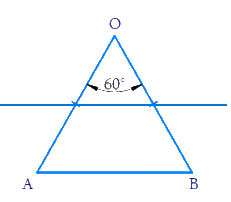\begin{align}\therefore \angle {{OAB}}\,= \,\angle {{OBA}} \end{align} (Angles opposite equal $$= \rm{}x^\circ$$ (say) sides in a triangle)

\begin{align}\angle {{AOB + }}\angle {{OAB + }}\angle {{OBA = 18}}{{{0}}^{{o}}}\end{align} (angle sum of triangle)

\begin{align}{{{60}^\circ } + x^\circ + x^\circ }&= {{{180}^\circ }}\\{2{{x^\circ}}} &= {{{180}^\circ } - {{60}^\circ }}\\x^\circ &= {\frac{{{{120}^\circ }}}{2}}\\x^\circ &= {{{60}^\circ }}\end{align}

\begin{align}&= \frac{\theta }{{{{360}^\circ }}} \times \pi {r^2}\\ &= \frac{{{{60}^\circ }}}{{{{360}^\circ }}} \times \pi {r^2}\end{align}

\begin{align} &= {\frac{{{{60}^\circ }}}{{{{360}^\circ }}} \times \pi {r^2} - \frac{{{r^2}\sqrt 3 }}{4}}\\& = {\frac{{\pi {r^2}}}{6} - \frac{{{r^2}\sqrt 3 }}{4}}\end{align}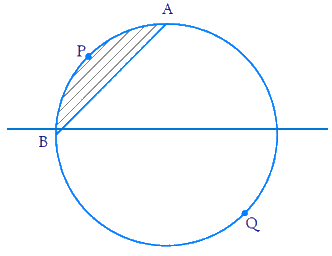\begin{align}= \pi {r^2} \end{align} - $$\text{Area of minor segment}$$

Steps: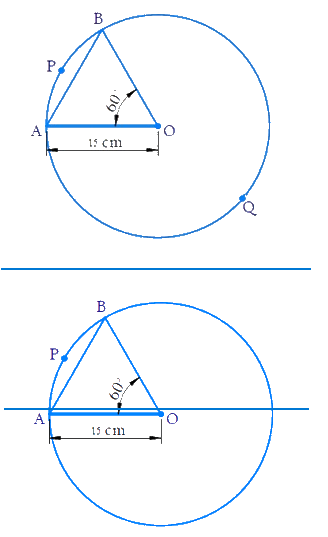Here,\begin{align}\rm{Radius, }r = 15\,cm\,\,\,\,\,\,\,\,{\rm{\theta }} = 60^\circ\end{align}

Area of the sector OAPB \begin{align}= \frac{{\rm{\theta }}}{{{{360}^{\rm{\circ}}}}} \times \pi {r^2}\end{align}

\begin{align}& = {\frac{{{{60}^\circ }}}{{{{360}^\circ }}} \times \pi {r^2}}\\&= { \frac{1}{6} \times 3.14 \times {{15}^2}}\\& = {117.75\,\rm{{c}}{{{m}}^2}}\end{align}

In $$\Delta \,{{OAB}},$$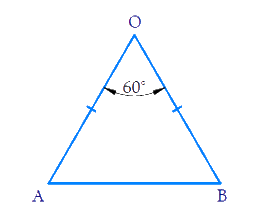\begin{align}OA = OB = r\,\,\,\,\,\,\,\,\,\,\,\,\,\,{\rm{}}\left( {{\text{radii of the circle}}} \right)\end{align}

\begin{align} \angle OBA=\angle OAB \end{align} ( Angles opposite equal sides in a triangle)

\begin{align} \angle {AOB+}\angle {OBA+}\angle {OAB}&=180^\circ\\text{Angle sum of a } &\text{triangle} ) \\\angle {OBA\!+\!}\angle {OAB}&\!=\!8{{{0}}^{{o}}}\!-\!{60^\circ} \\ {2}\angle {OAB}&=120^{\circ} \\ \angle {OAB}&={{60}^{\circ }} \end{align} \(\therefore \,\Delta {{OAB}} is an equilateral triangle (as all its angles are equal)

$$\text{Area of }\Delta AOB$$\begin{align}&= \frac{{\sqrt 3 }}{2}{({{side}})^2}\\& = \frac{{\sqrt 3 }}{2}{r^2}\\& = \frac{{\sqrt 3 }}{4}{(15)^2}\\& = \frac{{225\sqrt 3 }}{4}\\ &= 56.25\sqrt 3 \\ &= 56.25 \times 1.73\\& = 97.3125\,\rm{{ cm}^2}\end{align}

Area of minor segment $$APB =$$ Area of sector $$OAPB\,-\,$$Area of $$\Delta AOB$$

\begin{align}&= 117.75 - 97.3125\\ &= 20.4375\,\rm{{c}}{{{m}}^2}\end{align}

Area of major segment $$AQB\,=\,$$ Area of circle $$-\,$$Area of minor segment $$APB$$

\begin{align}&= \pi {(15)^2} - 20.4375\\&= 3.14 \times 225 - 20.4375\\&= 706.5 - 20.4375\\&= 686.0625\,\rm{{ cm}}^2 \end{align}

## Chapter 12 Ex.12.2 Question 7

A chord of a circle of radius $$12\, \rm{cm}$$subtends an angle of $$120^\circ$$ at the centre. Find the area of the corresponding segment of the circle.

(Use $$\pi =3.14$$ and $$\sqrt{3}=1.73$$)

### Solution

What is known?

A chord of a circle with radius $$({r}) = 12 \,\rm{cm}$$ subtends an angle $$(\theta) =120^\circ$$ at the centre.

What is unknown?

Area of segment of the circle.

Reasoning:

In a circle with radius r and angle at the center with degree measure $$\theta$$;

(i) Area of the sector\begin{align} = \frac{{\rm{\theta }}}{{360}^{\rm{o}}} \times \pi {r^2}\end{align}

(ii) Area of the segment = Area of the sector - Area of the corresponding triangle

Draw a figure to visualize the problem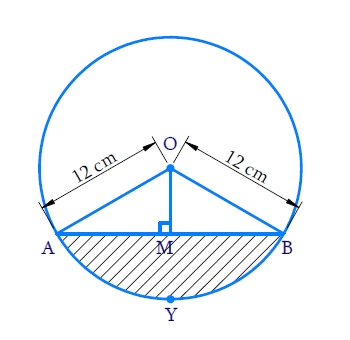Here,\begin{align}{\rm{Radius,}}r=12\rm{cm}\,\,\,\,\,\,{\rm{\theta }} = {120^{\rm{\circ}}}\end{align}

Visually it's clear from that figure that;

AB is the chord subtends$${120^{\rm{\circ}}}$$ angle at the centre.

To find area of the segment AYB, We have to find area of the sector OAYB and area of the$$\Delta AOB$$

(i) Area of sector OAYB \begin{align}= \frac{{\rm{\theta }}}{{{{360}^\circ }}}{\rm{ \times }}\pi {r^2}\end{align}

(ii) Area of  \begin{align}\Delta {\rm{AOB}} = \frac{1}{2} \times \rm{}base \times \rm{}height\end{align}

For finding area of$$\Delta {AOB,}$$draw $${OM}\, \bot\, {AB}$$then find $${AB}$$ and height$${OM.}$$

\begin{align}\Delta {{OMA}}\,& = \,\Delta {{OMB = 9}}{{{0}}^ \circ }\end{align}

$$\therefore \;\Delta \,{{OAM}}\, \cong \Delta \, {OBM}$$(By RHS Congruency)

$$\Rightarrow \quad \angle {{AOM}}= \angle {{BOM}}$$\begin{align} &= \frac{1}{2}\angle {{AOB}}\\& = \frac{1}{2} \times {120^ \circ }\\ &= {60^ \circ }\end{align}

\begin{align}\frac{AM}{OA}=\sin {{60}^\circ } = \frac{\sqrt 3 }{2}\end{align} \begin{align}& ={ AM = \frac{{OA\sqrt 3 }}{2}}\\ &= \frac{{12\sqrt 3 }}{2}\\ &= 6\sqrt 3 \end{align}

\begin{align}{\frac{{OM}}{{OA}}} {= \cos {{60}^\circ } = \frac{1}{2}}\end{align} \begin{align}OM& = \frac{{12\,\rm{{cm}}}}{2}\\&= 6\,\rm{{cm}}\end{align}

$$\text{Area of}\Delta OAB$$\begin{align}&=\frac{{{1}}}{{{2}}}\,\times \,{{base}}\,\times\,{{height}}\\&=\,\frac{{{1}}}{{{2}}}\times\,{{AB}}\, \times \,{{OM}}\end{align}

Steps:Here,\begin{align}{\rm{Radius, }}r = 12\,cm\,\,\,\,\,\,{\rm{\theta }} = {120^{\rm{\circ}}}\end{align}

$$\text{Area of the sector}$$\begin{align}&= \frac{{{{120}^\circ }}}{{{{360}^\circ }}} \times \pi {r^2}\\ &= \frac{1}{3} \times 3.14 \times {(12)^2}\\& = \frac{{452.16}}{6}\,\rm{cm^2}\\ &= 150.72\,\rm {c m^2}\end{align}

Draw a perpendicular $${OM}$$ from $${O}$$ to chord $${AB}$$

In $$\Delta \,{AOM}$$and $$\Delta \,{BOM}$$

$${AO}={BO}$$(radii of circle)

$${OM} = {OM}$$(common)

$$\angle {OMA} = \angle {OMB = {{90}^\circ}}$$

$$\therefore \;\Delta {AOM} \cong \Delta {BOM}$$(By RHS Congruency)

\begin{align}\therefore \angle {AOM} &= \angle {BOM} \\&= \frac{1}{2} \angle {AOB} = 60^\circ \end{align}

In $$\Delta {AOM}$$

\begin{align}\frac{{{{AM}}}}{{{{OA}}}}\, &=\,{{sin}}\,{{6}}{{{0}}^{{o}}}\\{{AM}}\, &= \,{{OA}}\frac{{\sqrt {{3}} }}{{{2}}}\\&=\frac{{{{12}}\sqrt {{3}} }}{{{2}}}\\&= 6\sqrt {{3}}\,\rm{{cm}}\\{AM} &= {2AM}\\&= 12\sqrt {{3}} \,\rm{{cm}}\end{align}

Area of $$\Delta \,{OAB}$$

\begin{align}&=\frac{1}{2}\times AB\times OM \\&=\frac{1}{2}\times 12\sqrt{3}\times 6 \\&=36\sqrt{3} \\ &=36\times 1.73 \\&=62.28\,\rm{c}{{{m}}^{2}} \\\end{align}

Area segment $${APB} =$$Area of sector $${OAPB}$$$$-$$Area of$$\Delta \,{OAB}\,$$

\begin{align}&=150.72-62.28\\&=88.44\; \rm{cm^2} \end{align}

## Chapter 12 Ex.12.2 Question 8

A horse is tied to a peg at one corner of a square shaped grass field of side $$15\,\rm{m}$$ by means of a $$5 \,\rm{m}$$ long rope (see given figure). Find

(i) the area of that part of the field in which the horse can graze.

(ii) the increase in the grazing area if the rope were $$10 \,\rm{m}$$ long instead of $$5\, \rm{m.}$$

(Use $$\pi = 3.14$$)

### Solution

What is known?

Length of the side of the square grass field  $$= 15 \,\rm{m}$$ and rope of length $$= 5\,\rm{m}$$  by which a horse is tied to a peg at one corner of the field.

What is unknown?

(i) Area of the field the horse can graze

(ii) Increase in grazing area if the rope were $$10\,\rm {m}$$ long instead of $$5 \,\rm{m}$$

Reasoning:

(i) From the figure it’s clear that the horse can graze area of a a sector of a circle with radius$$\rm{}(r)$$ $$\rm{}5\,m$$and angle with degree measure $${90^o}$$ (as the peg is at corner of a square and angle of square $$= {90^o}$$) length of the rope  $$= 5\,\rm{m}$$

$$\therefore \quad$$Area of a horse can graze $$=$$ Area of a sector

\begin{align}&= \frac{\theta }{{{{360}^\circ }}} \times \pi {r^2}\\&= \frac{{{{90}^\circ }}}{{{{360}^\circ }}} \times \pi {r^2}\\&= \frac{{\pi {r^2}}}{4}\end{align}

(ii) Similar to the first part

Area that can be grazed by the horse when length of the rope is $$\rm{}10\,m$$ is area of a sector of a circle with radius(r1) 10m and angle with degree measure $$\rm{}90^\circ$$

Area of the field horse can graze = Area of the sector

\begin{align} &= \frac{{{{90}^\circ }}}{{{{360}^\circ }}} \times \pi r_1^2 \\&= \frac{{\pi {r_1}^2}}{4} \end{align}

Increase in grazing area

\begin{align}& = \frac{{\pi r_1^2}}{4} - \frac{{\pi {r^2}}}{4}\\ &= \frac{\pi }{4}\left( {r_1^2 - {r^2}} \right)\end{align}

Steps: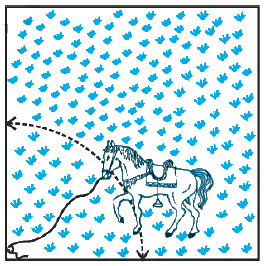(i) Area of the field the horse can graze = Area of sector of \begin{align}90^{\circ}\end{align} in a circle of radius $$5\,\rm{m}$$

\begin{align} &= {\frac{{{{90}^\circ }}}{{{{360}^\circ }}} \times \pi {{(5)}^2}}\\&={ \frac{{25}}{4} \times 3.14}\\&= \frac{{78.5}}{4}\\& = 19.625\,\rm{{{m}}^2}\end{align}

(ii) Area that can be grazed by the horse when rope is $$10\,\rm{m}$$ long.

\begin{align} &={\frac{{{{90}^\circ }}}{{{{360}^\circ }}} \times \pi {{(10)}^2}}\\&= {\frac{1}{4} \times 100 \times 3.14}\\&= \frac{{314}}{4}\\& = 78.5\,\rm{{{m}}^2}\end{align}

Increase in grazing area$$= 78.5{\rm{}m^2} - 19.625\,{\rm{}m^2} = 58.875{\rm{}m^2}$$

$$\therefore \quad$$Increase in grazing area \begin{align} &=(78.5-19.525)\, \rm{m}^{2} \\ &=58.875\, \rm{m}^{2} \end{align}

## Chapter 12 Ex.12.2 Question 9

A brooch is made with silver wire in the form of a circle with diameter $$35\,\rm{mm.}$$ The wire is also used in making $$5$$ diameters which divide the circle into $$10$$ equal sectors as shown in the figure. Find:

(i) The total length of the silver wire required.

(ii) The area of each sector of the brooch.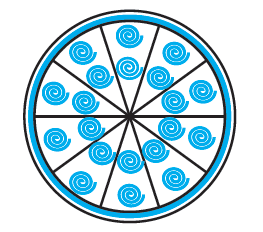### Solution

What is known?

A brooch is made with silver wire in the form of a circle with diameter $$35 \,\rm{mm.}$$ The wire is also used in making $$5$$ diameters which divides the circle into $$10$$ equal sectors.

What is unknown?

(i) The total length of silver were required

(ii) Area of each sector of the brooch

Reasoning:

(i) Since the silver wire is used in making the $$5$$ diameters and perimeter of the circular brooch.

$$\therefore$$ Total length of silver were required

$$=$$ Circumference of circle $$+ \,5\, \times\,$$diameter

$$= \pi {{d}} + 5{{d}}$$ Where $${d}$$ is diameter of the brooch

$$={d}\,(\pi + 5)$$

(ii) To find area of each sector of the brooch, we first find the angle made by each sector at the centre of the circle.

Since the wire divides into $$10$$ equal sectors

$$\therefore$$  Angle of sector \begin{align} \theta =\frac{360^\circ }{10^\circ } \end{align}

Radius of the brooch \begin{align}= {\frac{d}{2}} \end{align}

$$\therefore$$ Area of each sector of brooch \begin{align}= \frac{\theta }{{{{360}^\circ }}} \times \pi {r^2} \end{align}

Steps: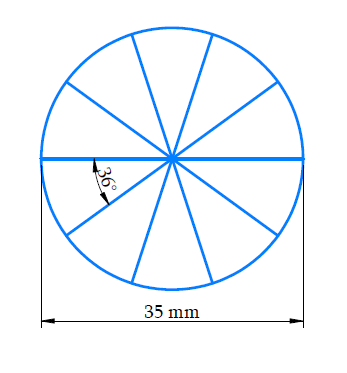(i) Diameter of the brooch $${(d) = 35 \,\rm{mm}}$$

Total length of silver wire required $$=$$ Circumference of brooch $$+\, 5\,$$ diameter

\begin{align}&= \pi d + 5d\\& = (\pi + 5)35\,\\ &= \left( {\frac{{22 + 35}}{7}} \right) \times 35\\ &= 57 \times 5\\ &= 285\,\,\rm{{mm}}\end{align}

ii) Radius of brooch \begin{align}\left( r \right) = \frac{{35}}{2} = 17.5\,\rm{{mm}}\end{align}

Since the wire divides the brooch into $$10$$ equal sectors

$$\therefore$$ Angle of sector \begin{align} \theta = \frac{{{{360}^\circ }}}{{{{10}^\circ }}}\,\, = {36^\circ } \end{align}

$$\therefore$$ Area of each sector of the brooch

\begin{align}&= \frac{{{{36}^\circ }}}{{{{360}^\circ }}} \times \pi {r^2}\\& = \frac{1}{{10}} \times \frac{{22}}{7} \times 17.5 \times 17.5\\& = 2.2 \times 2.5 \times 17.5\\ &= 96.25\,\rm{{m}}{{{m}}^2}\end{align}

## Chapter 12 Ex.12.2 Question 10

An umbrella has $$8$$ ribs which are equally spaced (see given figure). Assuming umbrella to be a flat circle of radius $$\text{45 cm,}$$ find the area between the two consecutive ribs of the umbrella.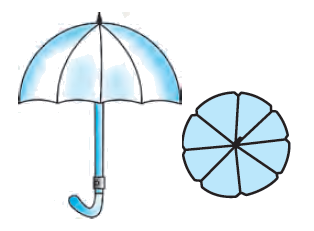### Solution

What is known?

An umbrella has $$8$$ ribs which are equally spaced. Assume umbrella to be a flat circle of radius $$= 45\,\rm{ cm.}$$

What is unknown?

The area between the $$2$$ consecutive ribs of the umbrella

Reasoning:

Since there are $$8$$ equal spaced ribs in an umbrella and the umbrella is assumed to be a flat circle.

$$\therefore \;$$Angle between $$2$$ consecutive ribs at the centre \begin{align}= \frac{{{{360}^o}}}{8} = {45^o}\end{align}

Area between $$2$$ consecutive ribs of the umbrella = Area of a sector with angle $$45^\circ$$

\begin{align}&= {\frac{\theta }{{{{360}^\circ }}} \times \pi {r^2}}\\& = {\frac{{{{45}^\circ }}}{{{{360}^\circ }}} \times \pi {r^2}}\\ &= {\frac{{\pi {r^2}}}{8}}\end{align}

Steps:

Since there are $$8$$ equally spaced ribs in the umbrella

$$\therefore$$ Angle between $$2$$ consecutive ribs $$\left( \theta \right)$$

\begin{align}&= \frac{{{{360}^\circ }}}{8}\\& = {45^\circ }\end{align}

Area between $$2$$ consecutive ribs of umbrella

\begin{align} &= {\frac{\theta }{{{{360}^\circ }}} \times \pi {r^2}}\\ &= {\frac{{{{45}^\circ }}}{{{{360}^\circ }}} \times \frac{{22}}{7} \times 45 \times 45}\\ &= {\frac{1}{8} \times \frac{{22}}{7} \times 45 \times 45}\\& = {\frac{{22275}}{{28}}{\text{c}}{{\text{m}}^2}}\\&{ = 795.535\,\,{\text{c}}{{\text{m}}^2}}\end{align}

## Chapter 12 Ex.12.2 Question 11

A car has two wipers which do not overlap. Each wiper has a blade of length $$\text{25 cm}$$ sweeping through an angle of $$115^\circ.$$Find the total area cleaned at each sweep of the blades.

### Solution

What is known?

A car has $$2$$ wipers which do not overlap. Each wiper has a blade length $$= 25 \,\rm{cm}$$ and sweeps through an angle $$\left( \theta \right) = {150^\circ}$$

What is unknown?

Total area cleaned at the sweep of the blade of the $$2$$ wipers.

Reasoning: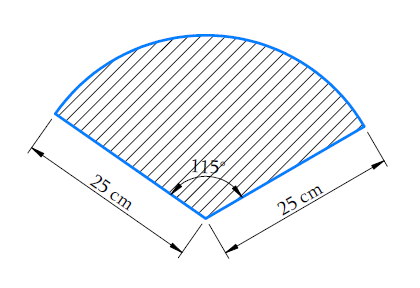Visually it is clear that

Area cleaned at the sweep of blades of each wiper $$=$$ Area of the sector with angle  $$115^\circ$$at the centre and radius of the circle $$\text{25 cm}$$

Since there are $$2$$ wipers of same blade length and same angle of sweeping. Also there is no area of overlap for the wipers.

$$\therefore \;$$Total area cleaned at each sweep of the blades $$=$$ \begin{align}2\ \times\end{align} Area cleaned at the sweep of each wiper.

Steps:

Area cleaned at the sweep of blades of each wiper = Area of the sector of a circle with radius $$\text {25 cm}$$ and of angle \begin{align}115^{\circ}\end{align}

\begin{align}& = \frac{\theta }{{{{360}^\circ }}} \times \pi {r^2}\\& = \frac{{{{115}^\circ }}}{{{{360}^\circ }}} \times \pi \times 25 \times 25\\& = \frac{{23}}{{72}} \times 625\pi \end{align}

Since there are $$2$$ identical blade length wipers

$$\therefore\;$$Total area cleaned at each sweep of the blades

\begin{align}& = 2 \times \frac{{23}}{{72}} \times 625\pi\\ & = 2 \times \frac{{23}}{{72}} \times \frac{{22}}{7} \times 625\\& = \frac{{23 \times 11 \times 625}}{{18 \times 7}}\\ &= \frac{{158125}}{{126}}\,{\text{c}}{{\text{m}}^{\text{2}}}\\ & = 1254.96\,\,\,{\text{c}}{{\text{m}}^{\text{2}}}\end{align}

## Chapter 12 Ex.12.2 Question 12

To warn ships for underwater rocks, a lighthouse spreads a red coloured light over a sector of angle $$80^\circ$$ to a distance of $$\text{16.5 km.}$$ Find the area of the sea over which the ships are warned.

(Use \begin{align}\pi=3.14\end{align})

### Solution

What is known?

A lighthouse spreads a red coloured light over a sector of angle of \begin{align}80^{\circ}\end{align} to a distance of $$16.5 \,\rm{km}$$ to warn ships for underwater rock.

(Use \begin{align}\pi=3.14\end{align})

What is unknown?

Area of the sea over which the ships are warned.

Reasoning:

Since the lighthouse spreads red coloured light over a sector  of a circle with radius $$\text{= 16.5 km}$$ and angle with degree measure \begin{align}80^{\circ}\end{align}.

Area of sea over which the ship are warned = area of the sector of the circle with radius 16.5km and angle with degree measure\begin{align}80^{\circ}\end{align}.

\begin{align}&={\frac{\theta}{360^{\circ}} \times \pi r^{2}} \\ &={\frac{80^{\circ}}{360^{\circ}} \times \pi r^{2}} \\ &={\frac{2}{9} \times \pi r^{2}} \end{align}

Steps:

Area of sea over which the ships are warned = Area of a sector of the circle with radius, $$\rm{}r=16.5\,km$$ and angle with degree measure\begin{align}80^{\circ}\end{align}.

\begin{align} &= \frac{{{{80}^\circ }}}{{{{360}^\circ }}} \times \pi {r^2}\\ &= \frac{2}{9} \times 3.14 \times 16.5 \times 16.5\\ &= 189.97\,\,{\text{k}}{{\text{m}}^2}\end{align}

## Chapter 12 Ex.12.2 Question 13

A round table cover has six equal designs as shown in figure. If the radius of the cover is $${28 \,\rm{cm},}$$ find the cost of making the designs at the rate of

$$\rm{₹}\, 0.35$$per $$\rm{cm^2.}$$(Use$$\sqrt{3}={ }1.7$$ )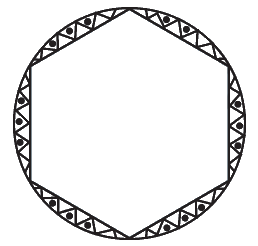### Solution

What is known?

A round table cover has $$6$$ equal designs as shown in the figure. The radius of the cover $$= 28\, \rm{ cm}$$and the rate of making design is $$\rm{₹\; 0.35}$$ per $$\rm{cm^2}$$

What is unknown?

The cost of making the design.

Reasoning:

In a circle with radius r and angle at the center with degree measure$$\theta$$

(i) Area of the sector\begin{align} = \frac{{\rm{\theta }}}{{{{360}^{\rm{o}}}}} \times \pi {r^2}\end{align}

(ii) Area of the segment = Area of the sector - Area of the corresponding triangle.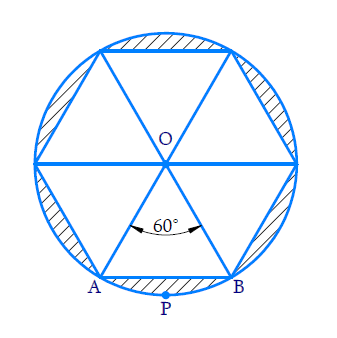(i) Visually it is clear that the designs are segments of the circle

$$\therefore\;$$Area of the design $$=$$ Area of $$6$$segments of the circle.

(ii) Since the table cover has $$6$$ equal design therefore angle of each segment at the center\begin{align} = \frac{{{{360}^o}}}{6} = {60^o}\end{align}

(iii) Consider segment $${APB.}$$Chord $${AB}$$ subtends an angle of $${60^\circ}$$ at the centre.

$$\therefore\;$$ Area of segment$${APB} =$$Area of sector $${AOPB}\, -$$ Area of $$\Delta {AOB}$$

(iv) To find area of $$\Delta AOB$$

In $$\Delta {AOB}$$

$${OA = OB}$$ (radii of the circle)

\begin{align}\angle {OAB} = \angle {OBA} \end{align} (angles oppositeequal sides of a triangle are equal)

\begin{align}\angle {AOB} + \angle {OAB} + \angle {OBA} = {180}^\circ\end{align} (Using angle sum property of a triangle)}

\begin{align}\angle AOB + 2\angle OAB &= {{180}^\circ }\\2\angle OAB &= {180^\circ } - {60^\circ }\\ \!\!&= 120^\circ \\ \angle OAB &= \frac{{120}}{2} \\&= {60^\circ }\\ &= \angle OBA\end{align}

Since all angles of a triangle are of measure $${60^\circ}$$

$$\therefore\;\Delta \,{AOB}$$is an equilateral triangle.

Using area of equilateral triangle \begin{align}=\frac{{\sqrt 3 }}{4}{\left( \rm{side} \right)^2}\end{align}

Area of\begin{align}\Delta AOB = \frac{{\sqrt 3 }}{4}{r^2}\end{align}

Area of sector \begin{align}{{AOBP = }}\frac{{{{6}}{{{0}}^{{\circ}}}}}{{{{36}}{{{0}}^{{\circ}}}}}\times \pi {{{r}}^{{2}}}\end{align}

Area of Segment APB \begin{align}{\rm{ = }}\frac{{{{60}^o}}}{{{{360}^o}}}\pi {r^2} - \frac{{\sqrt 3 }}{4}{r^2}\end{align}

Area of designs \begin{align}{\rm{ = 6}} \times \left( {\frac{{{{60}^o}}}{{{{360}^o}}}\pi {r^2} - \frac{{\sqrt 3 }}{4}{r^2}} \right)\end{align}

Since we know cost of making $$1\,\rm{cm}^2$$of designs we can use unitary method to find cost of designs.

Steps:From the figure we observe the designs are made in the segments of a circle.

Since the table cover has $$6$$ equal designs

$$\therefore\;$$angle subtended by each chord (bounding the segment) at the center \begin{align}= \frac{{{{360}^ \circ }}}{6} = {60^ \circ }\end{align}

Consider $$\Delta AOB$$

$$\Delta {OAB}\,=\,\Delta {OBA }$$($${QOB = OA,}$$angles opposite equal sides in a triangle are equal)

$$\Delta {AOB}\,{+}\,\Delta {OAB}\,{+}\,\,\Delta {OBA}\,{=18}{{{0}}^{{o}}}$$ (angle sum of a triangle)

\begin{align}{2}\Delta {OAB}\,&{=}\,{18}{{{0}}^{{o}}}-{6}{{{0}}^{{o}}} \\ \Delta {OAB}\,\,&{=}\,\frac{{12}{{{0}}^{{o}}}}{{2}} \\ & {=6}{{{0}}^{{o}}} \end{align}

$$\therefore\;\Delta {AOB}$$is an equilateral triangle

Area of $$\Delta AOB$$

\begin{align}&= \frac{{\sqrt 3 }}{4}{({\rm{side}})^2}\\ &= \frac{{\sqrt 3 }}{4} \times {(28)^2}\\ &= \sqrt 3 \times 7 \times 28\\& = 196\sqrt 3 \\ &= 196 \times 1.7\\& = 333.2\,\rm{cm}^2\end{align}

Area of sector $$OAPB$$

\begin{align}&=\,\frac{{{{6}}{{{0}}^{{o}}}}}{{{{36}}{{{0}}^{{o}}}}}{\times}\,{\pi }{{{r}}^{{2}}}\\\,&= \,\frac{{{1}}}{{{6}}}\,{\times }\,\frac{{{{22}}}}{{{7}}}\,{\times}\,{{28}}\,{\times }\,{{28}}\\\,\,& = \,\frac{{{{11} \times 4 \times 28}}}{{{3}}}\\\, &= \,\frac{{{{1232}}}}{{{3}}}\,\rm{{cm}^{{2}}}\end{align}

Area of segment $${APB =}$$ Area of sector $${OAPB}\, -$$Area of $$\Delta {AOB}$$

\begin{align} &= \left( {\frac{{1232}}{3} - 333.2} \right)\,\rm{cm^2}\end{align}

Area of designs $$= 6$$ Area of segment

\begin{align} &= 6 \times\!\!{ }\left( \frac{{1232}}{{3}}{-333}{.2} \right) \\ &= 2464 - 1999.2\,\rm{c}{{{m}}^{{2}}} \\ \\ &= 464{.8}\,\rm{c}{{{m}}^{{2}}} \end{align}

Cost of making $$1\, \rm{cm}^2$$of designs $$\rm{= ₹\; 0.35}$$

$$\therefore\;$$Cost of making $$464.8\,\rm {cm}^2$$ of designs

$$= \rm{₹}\,0.35 \times 464.8\\ =\rm{₹}\, 162.68$$

## Chapter 12 Ex.12.2 Question 14

Tick the correct answer in the following:

Area of a sector of angle $$p$$ (in degrees) of a circle with radius $${R}$$ is

(A) \begin{align}\frac{{P}}{180^{\circ}} \times 2 \pi {R}\end{align}

(B) \begin{align}\frac{{P}}{180^{\circ}} \times 2 \pi {R}^{2} \end{align}

(C) \begin{align}\frac{{P}}{720^{\circ}} \times 2 \pi {R}\end{align}

(D) \begin{align}\frac{{P}}{720^{\circ}} \times 2 \pi {R}^{2}\end{align}

### Solution

What is known?

A sector of angle $$p$$ (in degree) of a circle with radius $${R.}$$

What is unknown?

Area of a sector.

Reasoning:

Consider

Area of the sector of angle \begin{align}\theta =\frac{{ }\!\!\theta\!\!{ }}{{360}}{ }\!\!\times\!\!{ }\,\pi {{{r}}^{{2}}}\end{align}

where $${r}$$ is the radius of the circle

Here $${θ = p}$$ and $${r = R}$$

$$\therefore$$ Substituting above values in formula we get

Area of the sector \begin{align} = \frac{{{p}}}{{{{36}}{{{0}}^{{o}}}}}{\times \pi }{{{R}}^{{2}}}\end{align}

Multiplying numerator and denominator of formulas obtained above  by 2 we get

Area of the sector \begin{align} = \frac{{{p}}}{{{{72}}{{{0}}^{{o}}}}}{\times 2\pi }{{{R}}^{{2}}}\end{align}

Steps:

If radius of a circle $$= {R}$$

We know, Area of sector of angle \begin{align}= \,\frac{\theta }{{{{36}}{{{0}}^{{o}}}}}{ \times \pi }{{{R}}^{{2}}}\end{align}

$$\therefore$$ Area of sector of angle $$p$$

\begin{align} &= \frac{{{p}}}{{{{36}}{{{0}}^{{o}}}}}{ \times \pi }{{{R}}^{{2}}}\\ \,\,\,\,\,\,\,\,\,& = \frac{{{2}}}{{{2}}}\left( {\frac{{{p}}}{{{{36}}{{{0}}^{{o}}}}}{ \times \pi }{{{R}}^{{2}}}} \right)\\\, \,\,\,\,\,\,\,\,\, &= \frac{{{p}}}{{{{72}}{{{0}}^{{o}}}}}{ \times 2\pi }{{{R}}^{{2}}}\end{align}

Hence D is the correct answer.